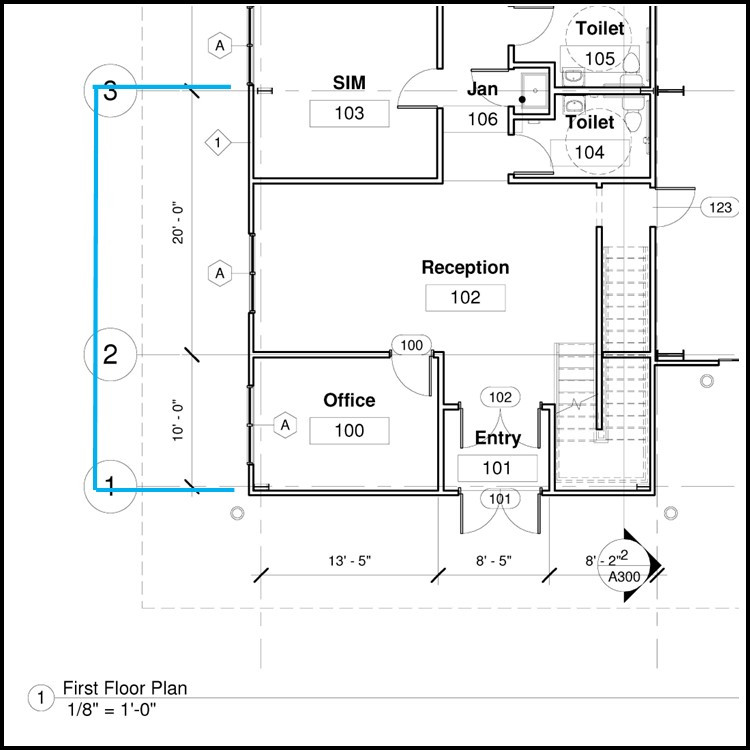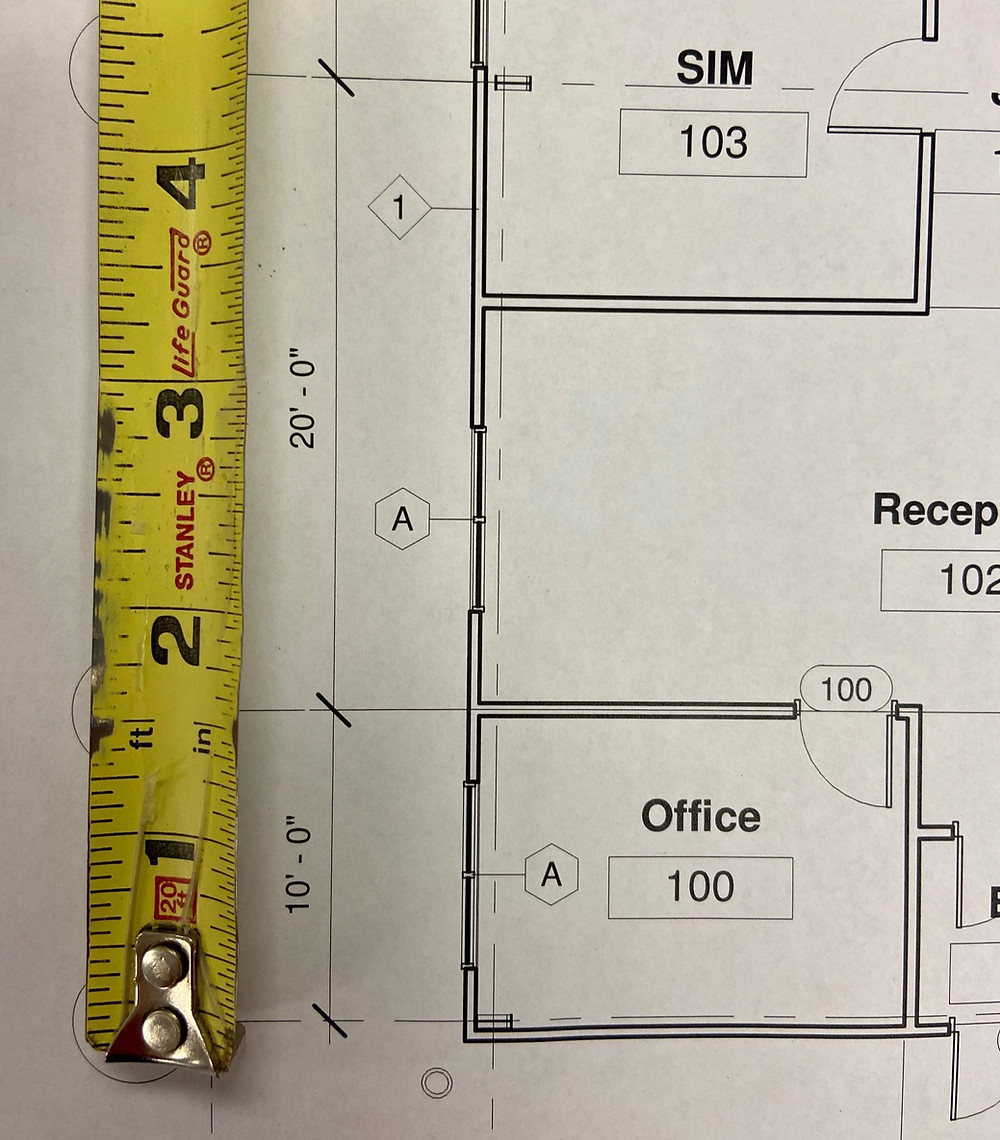Search

# Re-Scaling Construction Drawings

Updated: Jan 28

### A 4 Step Process on How to Scale Construction Drawings

Sometimes the drawings for a building project are not to scale, or they're at a scale that's inconvenient for those who are working with them.

In these situations it's beneficial to re-scale the drawings to better fit your needs.

For this example we'll be working with a drawing that has been enlarged from it's original 1/8 inch scaling (1/8 of an inch = 1 foot).

The method we'll use is: What you Want divided by What you Have = What you Need

At Digital Blueprint we use this method of re-scaling frequently when drawings have been enlarged or reduced making them out of scale.

### 1. Find an Object to Scale From - the Bigger the Better

To do this we need a printed copy of the drawing. It must be printed 1 to 1 with no shrinking or enlarging from the size it currently is. To start scaling you need to find something in the drawing that you can scale from. This needs to be an object or marking that you know the true measurement of. To make a more accurate scaling it's best to choose the largest measurement possible. If the drawing has called out measurements noted on it then you can choose any one of these. Usually a section of wall or a property line works best. We'll be using the 10 and 20 foot sections of wall in the picture below for a combined measurement of 30 feet.Sometimes drawings don't have measurements listed, so you have to get creative and find something that you know the dimensions of. This could be a part of a structure or a piece of building material. When all else fails, you can use a door frame since the industry standard for these is about 3 feet (assuming your looking at building's in the United States).

### 2. What You Have

Once you've found the object that you are going to scale from you need to measure it as accurately as possible. Ideally you will have the ability to measure it within a 32nd of an inch. Write this measurement on a piece of paper.In our example our combined section of walls measures 4 and 15/32 of an inch.

Next we need to turn our fraction into a decimal by dividing 15 by 32 which = 0.46875.

Now we have identified what we have: a 30 foot section of wall currently measuring 4.46875 inches.

### 3. What You Want

Next, you need to identify what you want. This is the measurement the object should be when it's in the scale you want. For our example we want our drawing to be at the 1/8 inch scale as it was originally intended to be: 1/8 of an inch = one foot.

If our drawing was scaled at 1/8 inch, each foot of our 30 foot wall would be 1/8 of an inch for a total of 3.75 inches.

We find this by turning our fraction (1/8) into a decimal by dividing 1 by 8 which = 0.75

Then we multiply 0.75 x 30 (the length of our wall section) which = 3.75 inches.

(*Alternatively if you needed 1/4 or 1/16 inch scale you would follow the same steps)

This tells us that our 30 foot section of wall should measure 3.75 inches when it's at 1/8 inch scale. Recall that currently our 30 foot wall is measuring 4.46875 inches, so it's larger than it should be.

### 4. What You Need

This step is fairly simple. We just need to divide our two numbers to get the percentage that will bring our drawing back to the scale we want.

What you Want divided by What you Have = What you Need

3.75 divided by 4.46875 = 0.8391608

0.8391608 is the number we need to multiply by to get our measurements to 1/8 inch scale.

In our example the 30 foot wall section currently measured 4.46875 inches. If we multiply this by 0.8391608 it gives us the accurate representation of 3.75 inches. Any other measurements we take from this drawing can now be multiplied by 0.8391608 to get the accurate sizing we need.

Alternatively, if you have access to a printer that allows enlargement and reduction by percentage, you can reprint your drawing to scale by turning the decimal 0.8391608 into a percentage. To do this simply move the decimal point two spaces to the right.

0.8391608 becomes 83.9%

If we print our current file at 83.9% it will then be to the 1/8 inch scale we want.

### Summary

To recap, here are the steps we followed:

1. Print your drawing one to one & find an object to scale from (no enlarging or reducing)

2. Measure the object as accurately as possible (what you have)

3. Calculate what length the object should be for the scale you want (what you want)

4. Divide what you want by what you have to get the percentage you need (what you need)

the measurement you want ÷ the measurement you have = the percentage you needRylee unlocks the mysteries of scaling for her new friend the butterfly.

556 views

See All